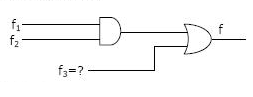# GATE | GATE CS 1997 | Question 40

Consider a logic circuit shown in figure below. The functions f1,f2 and (in canonical sum of products form in decimal notation) are :f1(w,x,y,z)
= ∑ 8,9,10
f2(w,x,y,z) = ∑ 7,8,12,13,14,15
f(w,x,y,z)  = ∑ 8,9

The Function f3 is

```a. ∑9,10
b. ∑9
c. ∑1,8,9
d. ∑8,10,15```

(A) a
(B) b
(C) c
(D) d